# True or false? Log-improbability is Shannon information

True or false? If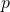is the probability of an event, then the Shannon information of the event is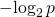bits.

I’m quite interested in knowing what you believe, and why you believe it, even if you cannot justify your belief formally.

Formal version. Let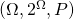be a discrete probability space with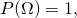and let event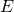be an arbitrary subset of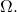Is it the case that in Shannon’s mathematical theory of communication, the self-information of the event is equal to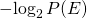bits?

## 84 thoughts on “True or false? Log-improbability is Shannon information”

1.Mung
Ignored
on said:

The quantity which a function f takes upon application to a given quantity.

2. Mung:
The quantity which a function f takes upon application to a given quantity.

Value

Well, hell. You’re right. They seem to be assuming numerical functions.

If you’ve had the least exposure to logic, then you’ve seen TRUE and FALSE (or T and F, or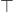and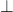) referred to as truth values.

Let me play the bookshelf trick — turnabout’s fair play. Lewis and Papadimitriou (1998), Elements of the Theory of Computation (2E):

If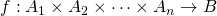is a function, and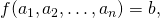where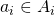for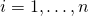and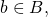then we sometimes call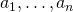the arguments of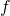and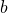the corresponding value of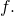Thusmay be specified by giving its value for each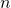-tuple of arguments.

(Emphasis in original.)

The Wikipedia article on value is inconsistent, indicating (correctly) in one bullet point that the value can be a number or any other mathematical object, and then restricting value to numbers in another bullet point.

It’s fine with me to refer to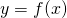as the image ofunder:

The word “image” is used in three related ways. In these definitions, f : X → Y is a function from the set X to the set Y.
Image of an element
If x is a member of X, then f(x) = y (the value of f when applied to x) is the image of x under f. y is alternatively known as the output of f for argument x.

(Emphasis added.) But that would give phoodoo conniptions.

I have discouraged computer science students from referring to “inputs” and “outputs” (and have never noticed the usage in a CS textbook) because I want them to be clear that a mathematical function is not the same thing as a subprogram of a computer program. However, it seems now, browsing the Web, that the preferred pedagogy in mathematics is “inputs” and “outputs.”

ETA: I’m getting to be pretty old.

3.Mung
Ignored
on said:

It’s because computers are taking over the world.

4. Mung:
It’s because computers are taking over the world.

Low-skilled Chinese workers, as little as they’re paid, are losing jobs to advanced automation. Manufacturing is on the rise in America, because highly automated manufacturing facilities are opening. Manufacturing jobs are not coming back to America — not the kind that blue-collar workers in the Rust Belt are qualified to do. “Believe me.”

5.Gordon Davisson
Ignored
on said:

Tomato Addict: Dembski equivocates, describing M*N*phi_S(T)*P(T|H) as a number, an upper bound, and a probability. He describes Log2 of this quantity as an Information Measure, which requires it to be a probability.

I disagree; for example, consider the Hartley information (aka Hartley function), which is the logarithm of the number of possibilities (ignoring their probability); or various measures of quantum information, which tend to involve logarithms of density matricies (which are related to probabilities, sort of…).

Also, there are plenty of legitimate meanings of “information” that have nothing to do with either logarithms or probabilities.

The upper bound on any probability is 1.0, but in an example he calculates a *negative* value for CSI, and therefore M*N*phi_S(T)*P(T|H) > 1.0, so it is neither a probability or an Information Measure.

Within the statistical theory of information, there’s a measure known as the information in the outcome (of one random variable, X) about another random variable (Y): I(X=x:Y) = H(Y) − H(Y|X=x). Intuitively, this is how much knowing that the variable X has the value x decreases your uncertainty about the value of Y. This can be negative! It’s entirely possible that H(Y|X=x) > H(Y), meaning that knowing that X=x increases your uncertainty about Y, and therefore has negative information about Y.

(Note: the expected value of this information, averaged over all possible values of X, is equal to the mutual information of X and Y, which is always non-negative.)

However, I also have a deeper objection here: this is a semantic quibble with the words Dembski uses to describe some of the quantities in his argument. Even if I thought it was a valid semantic argument, it still says nothing whatsoever about the logical validity of his argument. And that’s what really matters.

(Mind you, calling it “information” does take advantage of the prejudice that “information” sounds like it must have something to do with intelligence, and that’s the real reason he used the name, and that prejudice is both wrong and irrelevant. But it’s much better to face that bogus connotation head on than to quibble about the word he used.)

Further, M*N*phi_S(T)*P(T|H) is the WRONG probability. Dembski approximates the probability for the event T occurring exactly once, when it should be the probability of T occurring AT LEAST once. The result is that even when the upper bound is less than 1.0, is isn’t high enough, and therefore not an upper bound.

Hmm, I don’t understand this objection. M*N*phi_S(T)*P(T|H) is an upper bound on the expected number of times that an event matching a specification with at-least-that-low phi_S and at-least-that-low P. The expected number of such events is an upper bound on the probability of at least one such an event occurring.

(Note: the first “upper bound” in that paragraph is because it would be the expected number of such events if and only if there were exactly phi_S(T) other valid specifications with descriptions at least as short as T’s, and each of those other specifications had exactly the same probability under H as T. Realistically, neither of those conditions will be met, so the actual expected number of such events will be less.)

6.phoodoo
Ignored
on said:

Tom English: A coin does not have a tail, phoodoo. A coin does not have a head. Most coins have images of heads stamped into them. Some nickels have images of buffalo tails stamped into them.But coins don’t have heads and tails. So it’s imperative that you stop speaking of the outcomes of coin-flipping as heads and tails. It confuses everyone terribly when you call things what they’re really not, even though everyone agrees to call them that.

A coin is not really a coin, we just call it a coin.

THAT is your argument for why its ok to call something a value that is not a value?

I let others who are actually sane decide whose argument is more fucked up.

7.Mung
Ignored
on said:

Tom English: A coin does not have a tail, phoodoo. A coin does not have a head.

The ships I was on in the Navy had quite a few heads and a fantail.

Anyways, your response to phoodoo made me laugh. I’ll never think of a two-headed coin the same way again.

8.Mung
Ignored
on said:

Tom English: You know that the Shannon entropy is 1 bit for a fair coin toss. How do you get that result?

H = -(1/2 log 1/2 + 1/2 log 1/2)
H = -[(1/2)(-1) + (1/2)(-1)]
H = 1 bit per toss

: An Introduction to Information Theory
: Symbols, Signals and Noise
: John R. Pierce

D:\projects>irb
irb(main):001:0> Math.log2(1.0/2.0)
=> -1.0
irb(main):002:0> 1.0/2.0 * Math.log2(1.0/2.0)
=> -0.5
irb(main):003:0>

9.Gordon Davisson
Ignored
on said:

Tomato Addict: Again Gordon, I’m still trying to suss out your more subtle criticism.

“… this total probability turns out to be 1.”

Which probability? I don’t follow.

Yeah, trying to sort out which quantifications and hypotheticals are being summed over… is not easy to keep track of. The “total probability” I was talking about there is the total probability of at least one event occuring for which there exists a T_other for which the event matches T_other and for which phi_S(T_other)*P(T_other|H) <= phi_S(T)*P(T|H).

…that maybe didn’t help much, did it. Ok, let me turn it around and try from a different angle: I claim that there exist probability distributions and pattern-description languages such that for every event in the distribution, there exists a pattern T such that the event matches the pattern, and phi_S(T)*P(T|H) < 2^-500. This means that every event in the distribution has positive CSI.

How is this possible? The key is that my pattern-description language is not self-delimiting (aka not a prefix-free code), meaning that you can’t tell from the content of the description whether you’ve come to the end. Dembski gives the example of “four aces and the king of diamonds” as an example pattern, but “four aces” would also be a perfectly good pattern. My language happens to be binary, but that’s not actually that critical.

In my description language, the null (zero-bit-long) description matches an event that has a probability of 2^-501. Since phi_S(“”) = 1, phi_S(“”)*P(“”|H) = 2^-501 < 2^-500.

The two one-bit specifications “0” and “1” each match events with probability 2^-502, and these events are disjoint (with each other and the “” event), so we have phi_S(“0”) = phi_S(“1”) = 3 and phi_S(“0”)*P(“0″|H) = phi_S(“1”)*P(“1″|H) = 1.5*2^-501 < 2^-500. Note that the probability of an event matching one or the other of these specifications is 2 * 2^-502 = 2^-501.

There are four two-bit specifications, “00”, “01”, “10”, and “11”, each of which match disjoint events with probability 2^-503, so we have e.g. phi_S(T) = 7 and phi_S(T)*P(T|H) = 1.75*2^-501 < 2^-500. Again, the probability of an event matching one of the four is 4 * 2^-503 = 2^-501.

This pattern continues: for any 0 <= n <= 2^501, there are 2^n n-bit specifications, they each match disjoint events with probability 2^-(501+n), phi_S(T) = 2^(n+1)-1, phi_S(T)*P(T|H) < 2^-500, and the probability of an event matching some one of those specifications is 2^-501.

If you’ve been counting carefully, the total probability for all of those specifications is 1, meaning that they cover the event space. Every possible event matches some specification for which phi_S(T)*P(T|H) < 2^-500. Most of the specifications are impractically large (too large to actually write out within the visible universe), but like Graham’s number, they exist. And that means that an event with positive CSI is guaranteed to occur in a single trial.

(The probability that I made at least one obvious and embarrassing math mistake in the above is also near 1. I haven’t had enough coffee yet today.)

10. Tom English writes: I’ve published on the 2005 version of specified complexity. So I’m not raining on your parade alone when I say that the Evolutionary Informatics Lab seems to have committed to algorithmic specified complexity, which does have a clear interpretation as the log-ratio of two probability measures.

I’ve found a portion of your article online, but I’ll look harder. Please do keep sending the rain, it is spurring the discussion, and I am mostly waterproof. 🙂

Joe Felsenstein writes: “It is also a bit silly since it would accord a party-balloon a much higher “complexity” than a hummingbird.”

That’s my first good chuckle since the election. 🙂

11. Gordon, I was just writing a response to you earlier comment. I’ll come back for your recent comment later.

\\It’s entirely possible that H(Y|X=x) > H(Y), meaning that knowing that X=x increases your uncertainty about Y, and therefore has negative information about Y.\\

Careful!
H(Y) − H(Y|X=x) >= 0, always. For statistical variance, Shannon Information, and (I’m pretty sure) Kolmogorov Information, conditioning on another variable can only reduce the uncertainty. I may have misunderstood what you meant by negative information, so forgive me if I am correcting something you never intended to say.

\\Hmm, I don’t understand this objection. M*N*phi_S(T)*P(T|H) is an upper bound on the expected number of times that an event matching a specification with at-least-that-low phi_S and at-least-that-low P. The expected number of such events is an upper bound on the probability of at least one such an event occurring.\\

There is a quirk of Kolmogorov Information happening here: phi_S is an upper bound of compressibility, and to my understanding why Dembski terms the quantity an upper bound. The reason is there might always be some other coding scheme that would result in greater compression. We don’t know, nor do we care; Information Theorist happily work around the issue. I have no objection with this usage of “upper bound”.

My objection is the difference in probability between “T Happens Exactly Once” and “T Happens At Least Once”, and why Dembski’s bound is not high enough. Dembski approximates the former, but could have easily calculated the latter exactly.

That said, it may not exceed the true bound by very much; at the critical point where M*N* Phi_S(T)*P[T|H] = 0.5 I once worked out it is too small by a factor of Pi ~= 3.1416, so it’s within an order of magnitude in the range Dembski most cares about.

12. Gordon, a short(-ish) response to your recent comment — Maybe NOT so short … 😉

phi_S(T) is a STRANGE BEAST, and it took me a long time to figure it out. It is a cumulative function returning the total number of sequences that have coding length (compressed bits) less then or equal to the coding (bits) of T, for all sequence of the same length as T.

Phi_S(T) = 1 for the shortest coding of any sequence of length L. In any coding scheme, there is only one sequence such that Phi_S(T) = 1

Phi_S(T) = 2^L for all non-compressible sequences of length L. There are 2^(L-1) such sequences.

Phi_S(T) = 2^(L-1) for all sequences of length L that can be compress by exactly one bit. There are 2^(L-2) such sequences.

[ … ]

Phi_S(T) = 1 for the shortest coding of any sequence of length L. In any coding scheme, there is only one sequence such that Phi_S(T) = 1

*** Phi_S(T) returns a value equal to the number of sequences with coding length at least as short as that of T:: 1, 2, 4, 8, 16, …, 2^L

Now I’m self-taught at this level of IT, so I won’t claim certainty, but it should not be possible for every individual event to be CS. I’ll take a closer look at the rest of your comment, but I think this will all hinge on the above.

13.Mung
Ignored
on said:

Tomato Addict: That’s my first good chuckle since the election.

I’m still chuckling!

14. Tomato Addict: I’ve found a portion of your article online, but I’ll look harder.

Send me email (or PM here with your email address), and I’ll see if I can find the complete chapter. Most of what I omitted in the online version is history. I haven’t read the thing in forever. I’m sure I’d be unhappy with it now. I’ve learned a whole heap over the past 10 years.

15. Mung: H = -(1/2 log 1/2 + 1/2 log 1/2)
H = -[(1/2)(-1) + (1/2)(-1)]
H = 1 bit per toss

OK, so you summed over the possible outcomes, sometimes called atomic events, not all four of the events. The entropy is the expected value (probability-weighted average) of the specific entropy (the term I prefer to self-information) of the outcome of the coin toss. There are two specific entropy values, both equal to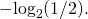I gave an example above for a loaded die. There are six outcomes, with probabilities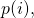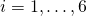, and thus six specific entropy values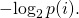Here, at last, is the crucial point: There are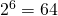events (subsets of the sample space). On each roll of the die, half of the events occur (contain the outcome). Say the outcome is 5. With 5 in the event, there aresubsets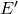of(leaving out 5), and for each of those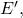the union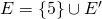is an event that occurs when the outcome is 5. You do not get to choose any of those 32 events that suits you, take it’s log-improbability, and call the result self-information (specific entropy). Only one of those events, the atomic event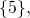enters into the calculation of entropy. Self-information (specific entropy) is defined for that atomic event, not the 31 events that contain 5 along with other possible outcomes.

What you have seen many times in ID is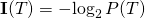for a target event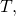with no “one event per outcome” restriction. Hopefully you have a better idea now of why that’s not Shannon self-information. It’s not related to how many bits a sender transmits to a receiver to communicate the occurrence of an event. The events are non-overlapping in Shannon’s mathematical theory of communication.

I’m tuckered. That’s about the best I’m going to do at the moment. If you’re still dubious, ask me a good question, and maybe I’ll come up with a good answer.

Well, I guess I can copy-and-paste another Ruby script I did yesterday afternoon. This one sums the log-improbabilities of all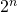events, withequiprobable outcomes, and reports it as “faux entropy.” I haven’t bothered to clean it up. Don’t give me grief.

 #!/usr/bin/ruby

 

n = ARGV.to_i print "Number of equiprobable possibilities: ", n, "\n" p = [1.0/n] * n print "Entropy: ", Math.log2(n), "\n" faux_entropy = 0.0 for i in 1..(n-1)     print "Events of size: ", i, "\n"     c = p.combination(i)     # print c.to_a, "\n"     for pp in c         prob_event = pp.inject(0) {|sum, px| sum + px}         log_improb_event = -Math.log2(prob_event)         print pp, " ", prob_event, " ", log_improb_event, "\n"         faux_entropy += prob_event * log_improb_event         print "Faux entropy (running total): ", faux_entropy, "\n"     end end print "Faux entropy (final result): ", faux_entropy, "\n" 

16. Previous script, hacked to do the calculations to for the loaded die that I designed a code for previously.

 #!/usr/bin/ruby # # Loaded die described in my comment on the first page # Entropy is compared to faux entropy #

 p = [1.0/16, 1.0/16, 1.0/4, 1.0/8, 1.0/2] # Omitting probability of 0 print "Entropy: " print p.inject(0) {|sum, px| sum - px * Math.log2(px)} print "\n" faux_entropy = 0.0 print "p.length: ", p.length, "\n" for i in 1..(p.length - 1)     print "Events of size: ", i, "\n"     c = p.combination(i)     # print c.to_a, "\n"     for pp in c         prob_event = pp.inject(0) {|sum, px| sum + px}         log_improb_event = -Math.log2(prob_event)         print pp, " ", prob_event, " ", log_improb_event, "\n"         faux_entropy += prob_event * log_improb_event         print "Faux entropy (running total): ", faux_entropy, "\n"     end end print "Faux entropy (final result): ", faux_entropy, "\n" 

ETA: Output
 Entropy: 1.875 p.length: 5 Events of size: 1 [0.0625] 0.0625 4.0 Faux entropy (running total): 0.25 [0.0625] 0.0625 4.0 Faux entropy (running total): 0.5 [0.25] 0.25 2.0 Faux entropy (running total): 1.0 [0.125] 0.125 3.0 Faux entropy (running total): 1.375 [0.5] 0.5 1.0 Faux entropy (running total): 1.875 Events of size: 2 [0.0625, 0.0625] 0.125 3.0 Faux entropy (running total): 2.25 [0.0625, 0.25] 0.3125 1.6780719051126376 Faux entropy (running total): 2.774397470347699 [0.0625, 0.125] 0.1875 2.415037499278844 Faux entropy (running total): 3.227217001462482 [0.0625, 0.5] 0.5625 0.8300749985576876 Faux entropy (running total): 3.6941341881511813 [0.0625, 0.25] 0.3125 1.6780719051126376 Faux entropy (running total): 4.218531658498881 [0.0625, 0.125] 0.1875 2.415037499278844 Faux entropy (running total): 4.671351189613664 [0.0625, 0.5] 0.5625 0.8300749985576876 Faux entropy (running total): 5.1382683763023635 [0.25, 0.125] 0.375 1.415037499278844 Faux entropy (running total): 5.66890743853193 [0.25, 0.5] 0.75 0.4150374992788438 Faux entropy (running total): 5.980185562991062 [0.125, 0.5] 0.625 0.6780719051126377 Faux entropy (running total): 6.403980503686461 Events of size: 3 [0.0625, 0.0625, 0.25] 0.375 1.415037499278844 Faux entropy (running total): 6.9346195659160275 [0.0625, 0.0625, 0.125] 0.25 2.0 Faux entropy (running total): 7.4346195659160275 [0.0625, 0.0625, 0.5] 0.625 0.6780719051126377 Faux entropy (running total): 7.858414506611426 [0.0625, 0.25, 0.125] 0.4375 1.1926450779423958 Faux entropy (running total): 8.380196728211224 [0.0625, 0.25, 0.5] 0.8125 0.2995602818589078 Faux entropy (running total): 8.623589457221588 [0.0625, 0.125, 0.5] 0.6875 0.5405683813627028 Faux entropy (running total): 8.995230219408446 [0.0625, 0.25, 0.125] 0.4375 1.1926450779423958 Faux entropy (running total): 9.517012441008244 [0.0625, 0.25, 0.5] 0.8125 0.2995602818589078 Faux entropy (running total): 9.760405170018608 [0.0625, 0.125, 0.5] 0.6875 0.5405683813627028 Faux entropy (running total): 10.132045932205466 [0.25, 0.125, 0.5] 0.875 0.19264507794239588 Faux entropy (running total): 10.300610375405062 Events of size: 4 [0.0625, 0.0625, 0.25, 0.125] 0.5 1.0 Faux entropy (running total): 10.800610375405062 [0.0625, 0.0625, 0.25, 0.5] 0.875 0.19264507794239588 Faux entropy (running total): 10.969174818604658 [0.0625, 0.0625, 0.125, 0.5] 0.75 0.4150374992788438 Faux entropy (running total): 11.280452943063791 [0.0625, 0.25, 0.125, 0.5] 0.9375 0.09310940439148147 Faux entropy (running total): 11.367743009680805 [0.0625, 0.25, 0.125, 0.5] 0.9375 0.09310940439148147 Faux entropy (running total): 11.455033076297818 Faux entropy (final result): 11.455033076297818 

17.Mung
Ignored
on said:

Hi Tom,

for … in is so not preferred Ruby syntax, lol!

But who cares, because Ruby is suppose to make programming fun.

And I see you’ve discovered the Range class.

well done

18.Mung
Ignored
on said:

Hi Tom,

For the indentation in your post did you use the html ampersand nbsp semicolon trick or did you find a better way?

19. Mung:
Hi Tom,

For the indentation in your post did you use the html ampersand nbsp semicolon trick or did you find a better way?

My last step in the editor was to do global search-and-replace of space with HTML non-breaking space. No big deal.

20. Mung:
Hi Tom,

for … in is so not preferred Ruby syntax, lol!

The problem is all of the logging (print statements). I’d have made it pure-applicative otherwise. That’s really my third Ruby script, produced in a rush. So…

21.Mung
Ignored
on said:

Don’t get me wrong. I’m impressed.

22. Tom – I’ll be in touch!
Thanks for the tip on the book too. At \$500 for the hardcover, I will opt for the Kindle edition!

I neglected your comment about the more recent Algorithmic Specified Information. I’ve seen this but haven’t studied it closely. It’s clear that Marks sat Dembski down and corrected the most stupid mistakes.

23. Gordon,
You had a comment about Hartley Information and other interpretations of Information, and I meant to respond. I don’t think this helps Dembski at all. If he rigorously defined CSI as he claimed, there should be be no need to discuss what he really means.

24. Tomato Addict: It’s clear that Marks sat Dembski down and corrected the most stupid mistakes.

I’m not sure that Dembski was much involved. Ewert’s doctoral dissertation (still sequestered at Baylor) was “Algorithmic Specified Complexity.” It is much, much easier to understand that Dembski (2005). I think it’s in large part a response to criticism of CSI in Gurevich and Passmore, “Impugning Randomness, Convincingly” (several versions online — be careful to get the latest).

25.Gordon Davisson
Ignored
on said:

Tomato Addict: I completely agree that Dembski’s measures (whatever you call them) aren’t rigorously defined. There are far too many sloppy bits, accounting loopholes, etc for it to have any real rigor.

26.Mung
Ignored
on said:

What does Faux Entropy measure?

27.phoodoo
Ignored
on said:

Mung,

Does a chocolate bunny have a head? Does a car have a tailgate?

I am not sure what his point is: EVERYTHING is what we call it.

Does that make a bit a value?

28. Mung, if this helps …

Information Theory says nothing about the value or content of the message. It’s an abstract way of describing all possible messages from a particular source. The meaning of any given message must be decided in advance by the sender and the receiver.

ex: “01100100 01101111 01100111” is the binary for the ACSII code that spells DOG. To understand the message we first need to agree on an alphabet, a language, and the connection that certain four-legged animals are dogs.

Note that some 2^24 message are possible in those 24 bits, but most of them won’t have any meaning in the English language.

29. Gordon: Sorry, I wasn’t sure what angle you were taking on Dembski. 🙂

Tom: Look for my email.

30.Mung
Ignored
on said:

Tomato Addict: Information Theory says nothing about the value or content of the message. It’s an abstract way of describing all possible messages from a particular source. The meaning of any given message must be decided in advance by the sender and the receiver.

The term “information” in information theory is used in a technical, mathematical sense not to be confused with the colloquial meaning of information. The “information” is not information about the message but rather it is information about the distribution of possible messages (or symbols).

31. Mung, I think you nailed it.

Tom, thanks for the Gurevich and Passmore article, that’s a good read. I have trouble accepting exclusionary inference in the first place; it impugns the assumptions as well as the randomness. The same is true for comparative inference, but the properties of these methods are well known, and the hypotheses compared are explicit – Perhaps that it my professional bias.

32. Mung: What does Faux Entropy measure?

I can’t make any sense of it. That’s more or less my point.

33.Mung
Ignored
on said:

Hi Tom,

If you had to describe Shannon entropy in plain English how would you go about it?

If I said Shannon entropy is an average would I be correct? But what is it an average of, or an average over?

Can you share any examples?

This site uses Akismet to reduce spam. Learn how your comment data is processed.